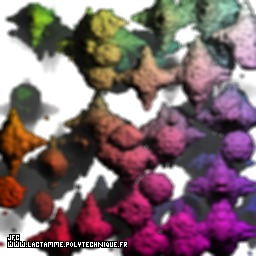The tridimensional John Conway's life game with random initial conditions -1.4% of occupied cells- [Le jeu de la vie tridimensionnel de John Conway avec des conditions initiales aléatoires -1.4% de cellules occupées-].

The bidimensional life game was initially defined by Conway. It uses an empty square mesh (all vertices are turned off). At time t=0 some vertices are occupied (they are turned on): this is the initial state. To go from the time t to the time t+1, it suffices to count for each vertex -or "Cell"- C(x,y) the number N of its neighbours (it is less than or equal to 3^2-1=8) and then to possibly change the state of M according to the following bidimensional automata rules:
```                    [R1 = Birth]        ((C(t).IS.off).AND.(N == 3))            ==> C(t+1) on
[R2 = Death]        ((C(t).IS.on).AND.((N < 2).OR.(N > 3))) ==> C(t+1) off
[R3]                other cases ==> C(t+1)=C(t)
```
The boundary conditions can be periodical or not.

This process can extended in a tridimensional space. The number N of neighbours of the vertex -or "Cell"- C(x,y,z) is computed (it is less than or equal to 3^3-1=26) and the preceding rules can be extended as follows:
```                    [R1 = Birth]        ((C(t).IS.off).AND.((N >= NB1).AND.(N <= NB2))) ==> C(t+1) on
[R2 = Death]        ((C(t).IS.on).AND.((N < ND1).OR.(N > ND2)))     ==> C(t+1) off
[R3]                other cases ==> C(t+1)=C(t)
```
The bidimensional and tridimensional processes can be extended one step further using two binary lists 'LD' and 'LA' ("Dead" -off- and "Alive" -on- respectively):
```                    [R1 = Birth]        ((C(t).IS.off).AND.(LD[N] == 1))  ==> C(t+1)=on
[R2 = Death]        ((C(t).IS.on).AND.(LA[N] == 1))   ==> C(t+1)=off
[R3]                other cases ==> C(t+1)=C(t)
```
("1" means "to change the state" and "0" means "the state is unchanged").

For this picture, the parameters have the following values:
```                    LD="000010001110000011100010000"
LA="111111100011111110000111111"
```

See some related pictures (including this one):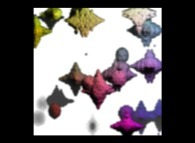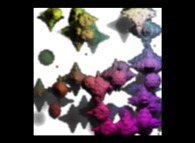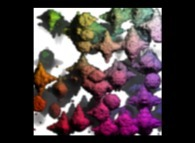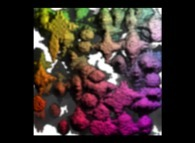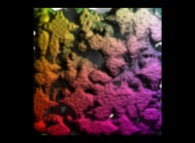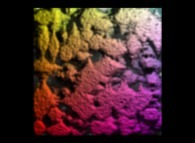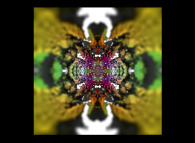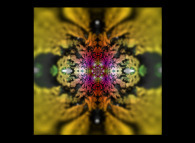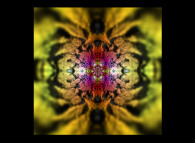(CMAP28 WWW site: this page was created on 03/12/2012 and last updated on 01/08/2022 15:15:10 -CET-)

[See all related pictures (including this one) [Voir toutes les images associées (incluant celle-ci)]]

[Please visit the related ImagesDesMathematiques picture gallery [Visitez la galerie d'images ImagesDesMathematiques associée]]

[Go back to AVirtualMachineForExploringSpaceTimeAndBeyond [Retour à AVirtualMachineForExploringSpaceTimeAndBeyond]]

[The Y2K Bug [Le bug de l'an 2000]]

[Site Map, Help and Search [Plan du Site, Aide et Recherche]]
[Mail [Courrier]]
[About Pictures and Animations [A Propos des Images et des Animations]]